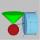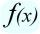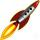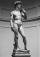# Derivation - math problems

#### Number of problems found: 25

• ConeInto rotating cone with dimensions r = 8 cm and h = 8 cm incribe cylinder with maximum volume so that the cylinder axis is perpendicular to the axis of the cone. Determine the dimensions of the cylinder.
• Sphere and coneWithin the sphere of radius G = 33 cm inscribe the cone with the largest volume. What is that volume, and what are the dimensions of the cone?
• DerivationExists a function whose derivation is the same function?
• FallThe body was thrown vertically upward at speed v0 = 79 m/s. Body height versus time describe equation h = v0 * t - (1)/(2) * 10 * t2. What is the maximum height body reach?
• StatueOn the pedestal high 4 m is a statue 2.7 m high. At what distance from the statue must the observer stand to see it at the maximum viewing angle? Distance from the eye of the observer from the ground is 1.7 m.

We apologize, but in this category are not a lot of examples.
Do you have an exciting math question or word problem that you can't solve? Ask a question or post a math problem, and we can try to solve it.

We will send a solution to your e-mail address. Solved examples are also published here. Please enter the e-mail correctly and check whether you don't have a full mailbox.